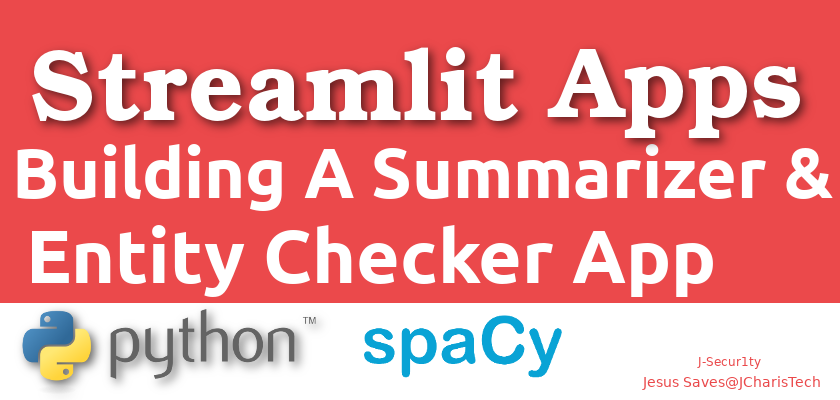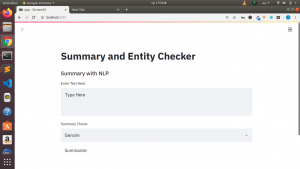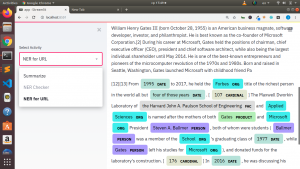# Summarizer and Named Entity Checker App with Streamlit and SpaCy

In this tutorial, we will be building a summarization app as well as a named entity checker app using streamlit, spacy,gensim and sumy. The NLP app will consist of three parts.

• Summarizer
• Entity Checker
• Entity Checker of Text Extracted from a URL

We will be using displacy from spacy to display our Named Entity in a nice html format on our front end.

Streamlit makes it quite easy to convert our python code into production ready applications. Let us see the basic idea and workflow of our app. The basic workflow of our app includes

1. Receiving Text Input From User using streamlit st.text_area() and st.text_input() functions
2. Summarizing our Received Text Using Gensim,Sumy and any other packages such as NLTK or SpaCy.
3. Extracting Text From a Given URL using BeautifulSoup and Urllib or Request
4. Entity Recognition using Spacy
5. Rendering our Extracted Named Entities using Displacy and Streamlit

This is how our final app will look likeLet us build our app.

First of all we will import our necessary packages

``````import streamlit as st

from gensim.summarization import summarize

# Sumy Summary Pkg
from sumy.parsers.plaintext import PlaintextParser
from sumy.nlp.tokenizers import Tokenizer
from sumy.summarizers.lex_rank import LexRankSummarizer

import spacy
from spacy import displacy

# Web Scraping Pkg
from bs4 import BeautifulSoup
from urllib.request import urlopen
``````

Then we will also have some individual functions to process our text, extract our entities and summarize our text.

``````
# Function for Sumy Summarization
def sumy_summarizer(docx):
parser = PlaintextParser.from_string(docx,Tokenizer("english"))
lex_summarizer = LexRankSummarizer()
summary = lex_summarizer(parser.document,3)
summary_list = [str(sentence) for sentence in summary]
result = ' '.join(summary_list)
return result

# Fetch Text From Url
@st.cache
def get_text(raw_url):
page = urlopen(raw_url)
soup = BeautifulSoup(page)
fetched_text = ' '.join(map(lambda p:p.text,soup.find_all('p')))
return fetched_text

@st.cache(allow_output_mutation=True)
def analyze_text(text):
return nlp(text)
``````

Our Main logic will be in a main function as below.

```def main():
"""Summaryzer Streamlit App"""

st.title("Summaryzer and Entity Checker")

activities = ["Summarize","NER Checker","NER For URL"]
choice = st.sidebar.selectbox("Select Activity",activities)

if choice == 'Summarize':
raw_text = st.text_area("Enter Text Here","Type Here")
summarizer_type = st.selectbox("Summarizer Type",["Gensim","Sumy Lex Rank"])
if st.button("Summarize"):
if summarizer_type == "Gensim":
summary_result = summarize(raw_text)
elif summarizer_type == "Sumy Lex Rank":
summary_result = sumy_summarizer(raw_text)

st.write(summary_result)

if choice == 'NER Checker':
raw_text = st.text_area("Enter Text Here","Type Here")
if st.button("Analyze"):
docx = analyze_text(raw_text)
html = displacy.render(docx,style="ent")
html = html.replace("\n\n","\n")
st.write(HTML_WRAPPER.format(html),unsafe_allow_html=True)

if choice == 'NER For URL':
raw_url = st.text_input("Enter URL Here","Type here")
text_preview_length = st.slider("Length to Preview",50,100)
if st.button("Analyze"):
if raw_url != "Type here":
result = get_text(raw_url)
len_of_full_text = len(result)
len_of_short_text = round(len(result)/text_preview_length)
st.success("Length of Full Text::{}".format(len_of_full_text))
st.success("Length of Short Text::{}".format(len_of_short_text))
st.info(result[:len_of_short_text])
summarized_docx = sumy_summarizer(result)
docx = analyze_text(summarized_docx)
html = displacy.render(docx,style="ent")
html = html.replace("\n\n","\n")
st.write(HTML_WRAPPER.format(html),unsafe_allow_html=True)

if __name__ == '__main__':
main()

```

To be able to render our NER in spacy we will be using displacy and wrap our result within an html as below.

`HTML_WRAPPER = """<div style="overflow-x: auto; border: 1px solid #e6e9ef; border-radius: 0.25rem; padding: 1rem">{}</div>"""`

Finally we will set unsafe_allow_html to True for our st.write() or st.markdown() to render our html text as valid html.CREDITS: Adrien Treuille from Streamlit , Innes from SpaCy and Johannes Baiter

You can check out the full video tutorial here

For more about building Machine learning Apps as well as NLP apps you can check this fascinating course